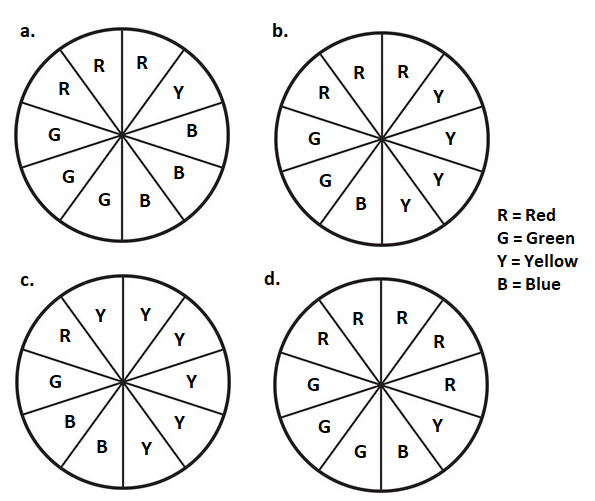# Probability

## Objective

Define probability and sample space. Estimate probabilities from experimental data.

## Common Core Standards

### Core Standards

?

• 7.SP.C.6 — Approximate the probability of a chance event by collecting data on the chance process that produces it and observing its long-run relative frequency, and predict the approximate relative frequency given the probability. For example, when rolling a number cube 600 times, predict that a 3 or 6 would be rolled roughly 200 times, but probably not exactly 200 times.

• 7.SP.C.7 — Develop a probability model and use it to find probabilities of events. Compare probabilities from a model to observed frequencies; if the agreement is not good, explain possible sources of the discrepancy.

?

• 7.RP.A.2

• 7.SP.A.1

## Criteria for Success

?

1. Understand the probability of an event can be represented as a fraction, where the numerator is the number of desired events and the denominator is the number of total events.
2. Perform an experiment and define the sample space; understand the sample space as a list of all possible outcomes for the experiment.
3. Estimate the probability of an event happening in an experiment.
4. Compare the accuracy of estimated probabilities from different experiments to the actual probability.

## Tips for Teachers

?

This lesson requires some prior preparation and materials (brown paper bags and different colored cubes) for Anchor Problem #1. See the notes in Anchor Problem #1 for further information.

### Lesson Materials

• Brown bag (2 per pair of students)
• Cubes (1 set per small group) — 15 with 5 of one color and 10 of different color(s)

### Remote Learning Guidance

If you need to adapt or shorten this lesson for remote learning, we suggest prioritizing Anchor Problem 2 (benefits from worked example). Find more guidance on adapting our math curriculum for remote learning here.

#### Fishtank Plus

• Problem Set
• Student Handout Editor
• Vocabulary Package

## Anchor Problems

?

### Problem 1

Give each pair or small group of students a brown bag of cubes, as described below in the notes.

Trial 1:

• Draw a cube from the bag and record the color. Replace the cube and shake the bag.
• Repeat the first step 4 more times for a total of 5 results.

Questions:

1. What do you think the sample space of the bag is based on your experiment so far?
2. Estimate the probability of pulling a red cube from the bag.

Trial 2:

• Draw a cube from the bag and record the color. Replace the cube and shake the bag.
• Repeat the first step 9 more times for a total of 10 results.

Questions:

1. Now what do you think the sample space of the bag is? Has your answer changed from Trial 1?
2. Estimate the probability of pulling a red cube from the bag. Has your answer changed from Trial 1? If so, how?

Trial 3:

• Draw a cube from the bag and record the color. Replace the cube and shake the bag.
• Repeat the first step 14 more times for a total of 15 results.

Questions:

1. Now what do you think the sample space of the bag is? Has your answer changed from Trials 1 and 2?
2. Estimate the probability of pulling a red cube from the bag. Has your answer changed from Trials 1 and 2? If so, how?

Check the bag:

• Look inside the bag and record the actual sample space.
• What is the actual probability of pulling a red cube from the bag?
• If you were to do a trial of 120 draws from the bag, about how many red cubes do you think you’d pull? Explain your answer.

### Problem 2

A spinner with different colors on it was spun 20 times. The data recording the color of each spin is shown below.

 Spin # Color Spin # Color 1 Red 11 Yellow 2 Green 12 Yellow 3 Green 13 Yellow 4 Yellow 14 Green 5 Blue 15 Red 6 Yellow 16 Red 7 Red 17 Yellow 8 Yellow 18 Green 9 Green 19 Red 10 Red 20 Yellow
1. What is the sample space of the spinner?
2. Does it seem that each color outcome is equally likely? Explain your reasoning.
3. Estimate the probability of spinning each color on the spinner.

Four spinners are shown below. Which spinner is most likely the spinner used for the data above?## Problem Set

?The following resources include problems and activities aligned to the objective of the lesson that can be used to create your own problem set.

?

Each of the 20 students in Mr. Anderson’s class flipped a coin ten times and recorded how many times it came out heads.

a.   How many heads do you think you will see out of ten tosses?

b.   Would it surprise you to see 4 heads out of ten tosses? Explain why or why not.

c.   Here are the results for the twenty students in Mr. Anderson’s class. Use this data to estimate the probability of observing 4, 5, or 6 heads in ten tosses of the coin. (It might help to organize the data in a table or in a dot plot first.)

 Student 1 2 3 4 5 6 7 8 9 10 11 12 13 14 15 16 17 18 19 20 # of heads 3 5 4 6 4 8 5 4 9 5 3 4 7 5 8 6 3 6 5 7